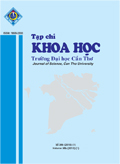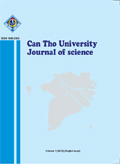Hướng dẫn
Đăng nhập
Tìm kiếm nâng cao

Tên bài báo
Tác giả
Năm xuất bản
Tóm tắt
Lĩnh vực
Phân loại
Số tạp chí

Bản tin định kỳ
Báo cáo thường niên
Tạp chí khoa học ĐHCT
Tạp chí tiếng anh ĐHCT
Tạp chí trong nước
Tạp chí quốc tế
Kỷ yếu HN trong nước
Kỷ yếu HN quốc tế
Book chapter
Bài báo - Tạp chí
Vol. 54, No. 5 (2018) Trang: 72-76
Tải về
 Article info. ABSTRACT Received 07 Dec 2017 Revised 20 Apr 2018Accepted 20 Jul 2018 A ground set of n elements and a class of its subsets, also known as feasible solutions, is given.  Moreover, each element in the ground set is associated a positive weight. In the setting of the original combinatorial optimization problem, each feasible solution corresponds to an objective value, often measured under the sum or the max of all element weights in the underlying solution. This paper is to address the problem of modifying the weight of elements in the ground set such that a prespecified subset becomes the  maximizer with respect to new weights and the cost is minimized. This problem is called the inverse version of the  maximization combinatorial optimization. Two quadratic algorithms were developed to solve this problem with sum objective function under Chebyshev norm and the bottleneck Hamming distance. Additionally, if the objective function is the max function then this problem can be solved in  time. Keywords Chebyshev norm, hamming distance, inverse optimization, maximization Cited as: Quoc, H.D., Kien, N.T., Thuy, T.T.C., Hai, L.H. and Thanh, V.N., 2018. Inverse version of the kth maximization combinatorial optimization problem. Can Tho University Journal of Science. 54(5): 72-76.

CTUJoS indexed by Crossref

Vietnamese | English

BC thường niên 2018Con số ấn tượng (VN | EN)Bản tin ĐHCTTCKH tiếng ViệtTCKH tiếng Anh

Vui lòng chờ...